##### Linear Algebra For Dummies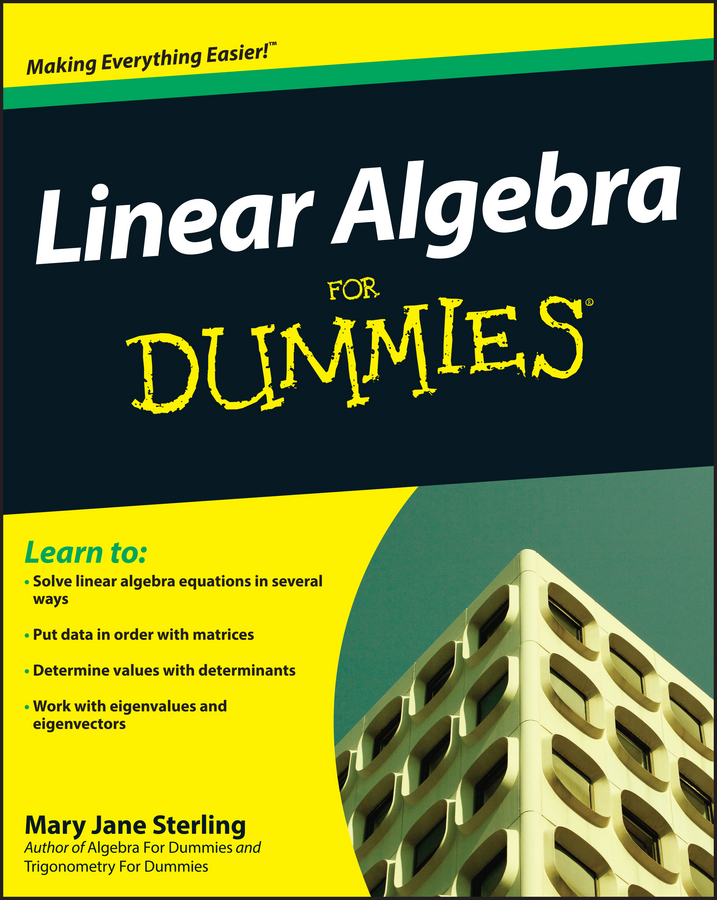Dividing variables in an algebra problem is fairly straightforward. Each variable is considered separately. The number coefficients are reduced the same as in simple fractions. When dividing variables, you write the problem as a fraction. Then, using the greatest common factor, you divide the numbers and reduce. You use the rules of exponents to divide variables that are the same — so you subtract the powers.

Example: Four friends decided to collect aluminum cans for recycling (and money). They collected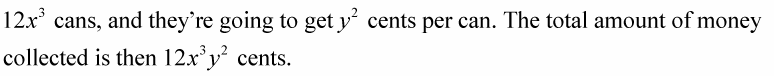• How will they divide the money?

Divide the total amount by four to get the individual amount that each of the four friends will receive. The only thing that divides is the coefficient.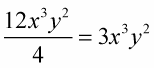• What is the total number of cans that each friend will be paid for?

Divide the total amount by four times the price per can.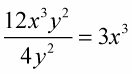Using variables is better than using just numbers because if the numbers change, then you still have all the shares worked out. Just let the x and y change in value.

These examples show how to divide using variables, coefficients, and exponents:

Example 1: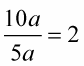Only the coefficients divide.

Example 2: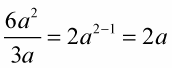Divide the coefficients, and divide the variables. Using the law of exponents, you divide the variables by subtracting the powers.

Some people prefer to write the answer with x in the denominator and a positive exponent rather than in the numerator with a negative exponent, but you can do it either way. In these examples, positive exponents are used:

Example 3: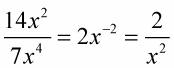Divide the coefficients, and divide the variables. Using the law of exponents, you divide the variables by subtracting the powers. Subtracting the powers leads to negative exponents, so you can write it as a fraction so that you have positive exponents.

Example 4: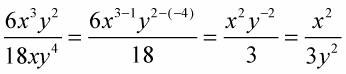Divide the coefficients, and divide the variables. Using the law of exponents, you divide the variables by subtracting the powers.